# Python结合Tableau，万字长文搞定传统线下连锁店数据分析

##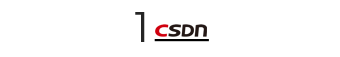## 分析背景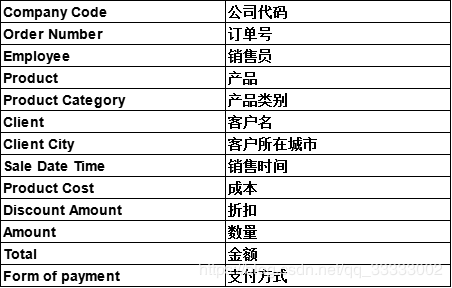## 分析框架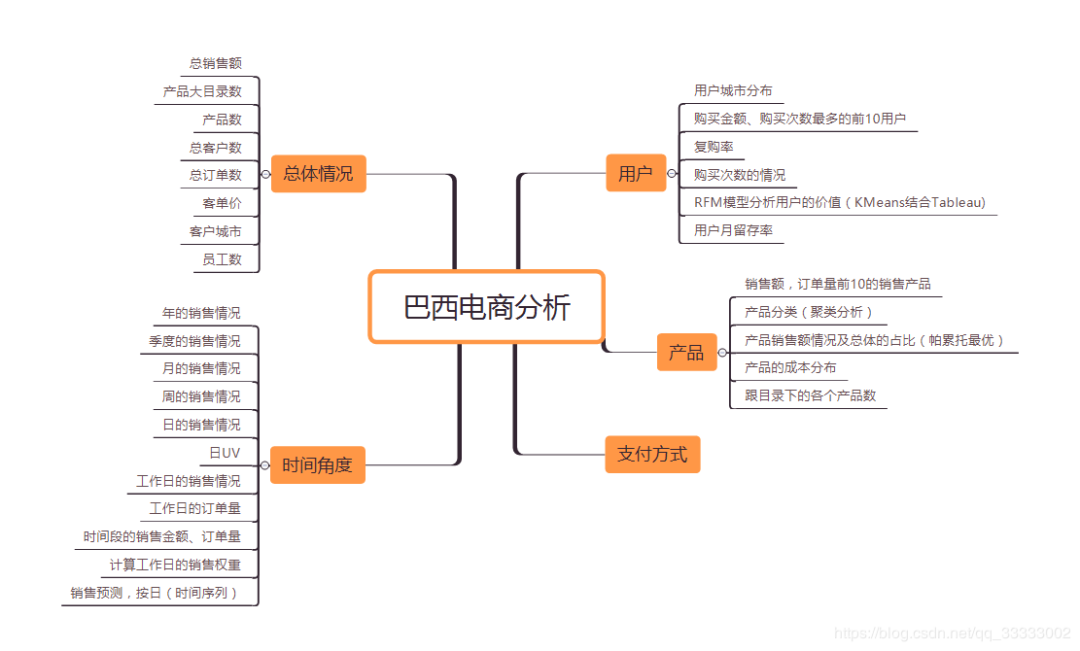## 数据清洗

### 3.1 读取数据，看看总体情况

# 导入相关包
import numpy as np
import pandas as pd

# 读取数据，设置分割符号
file_path = r'F:\ales Report.csv\Sales Report.csv'
data = df.get_chunk(30000000)
data.info()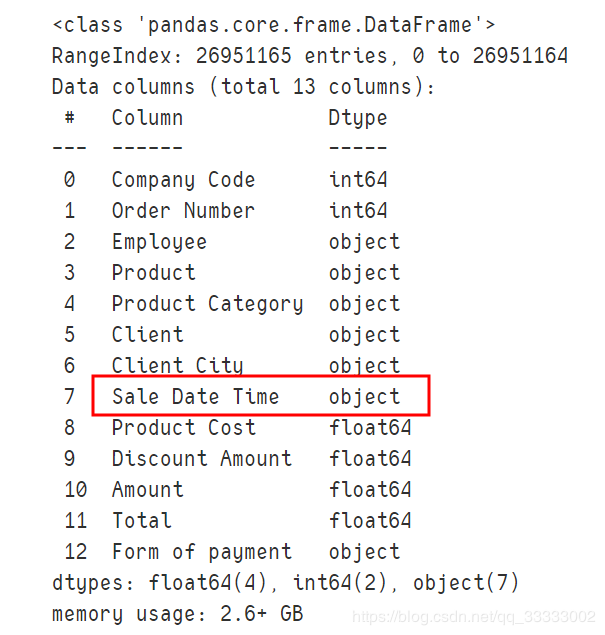# 查看NULL的数据：
data.isnull().sum()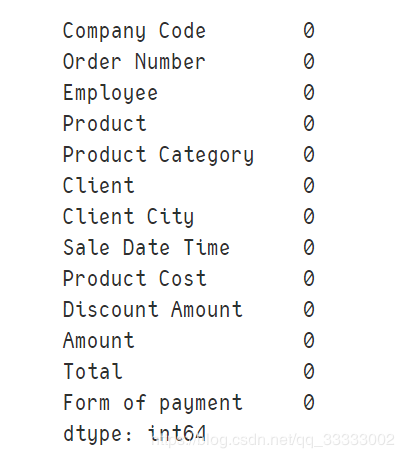查看数据的标准差，最大，最下值这些：
data.describe()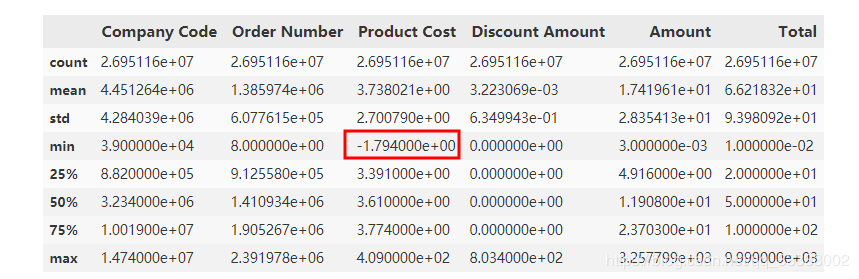data[data['Product Cost'] <= 0]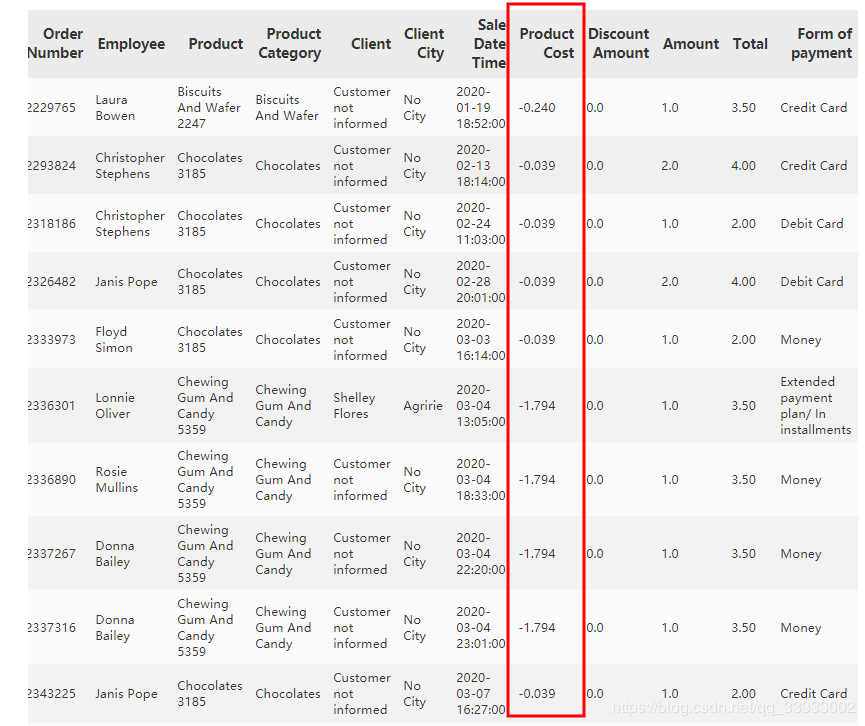len(data[data['Product Cost'] < 0])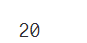data.drop(index=data[data['Product Cost'] < 0].index, inplace=True)



### 3.2 删除重复的数据

# 数据清洗，这里有489567条数据是重复的，删除这些数据
data[data.duplicated()]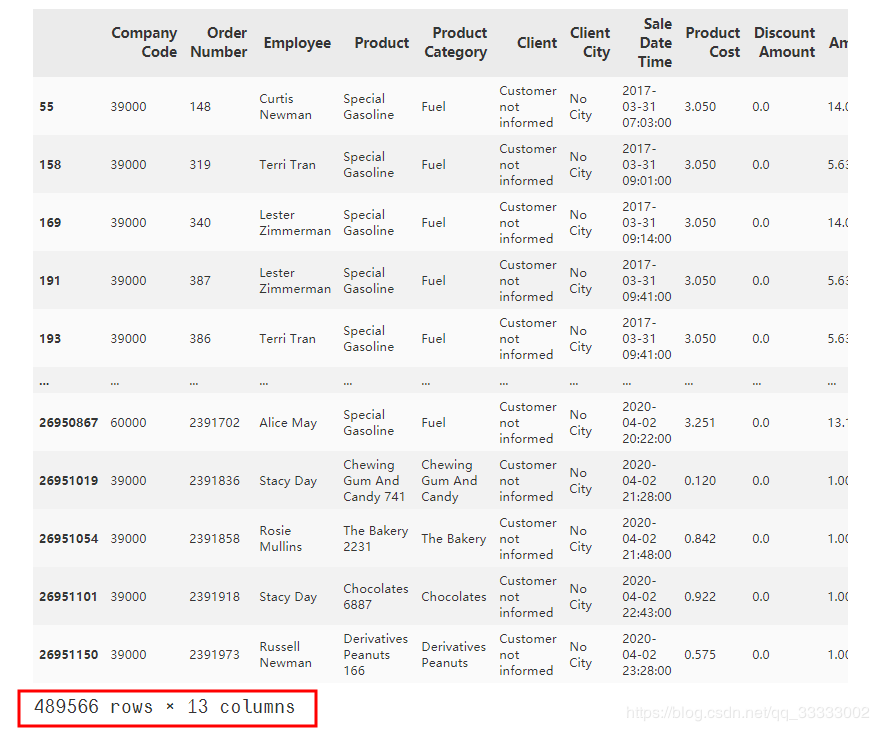# 删除重复的数据
# 这里的重复的数据是完全重复的，所有的值都是相同的，
# 这里只能判断为异常数据，直接删除掉
data.drop(index=data[data.duplicated()].index, inplace=True)



### 3.3 日期转换格式

data['Sale Date Time'] = pd.to_datetime(data['Sale Date Time'])
data.info()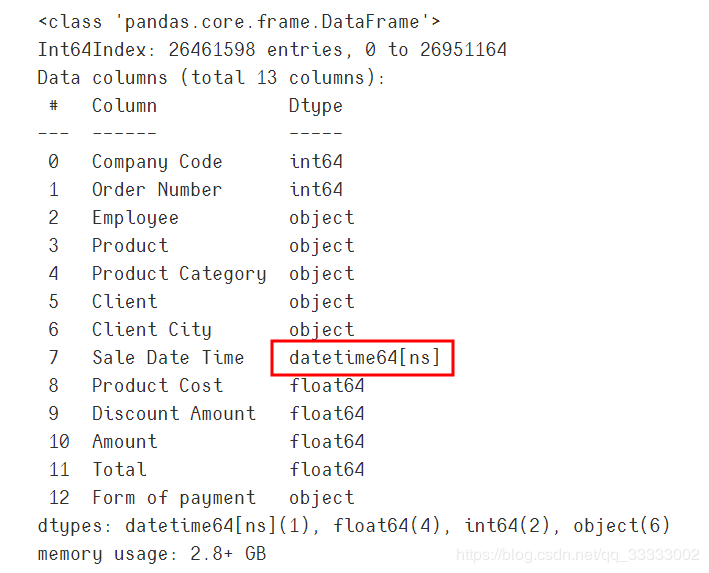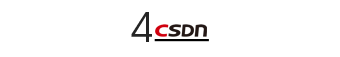## 分析

### 4.1 总体情况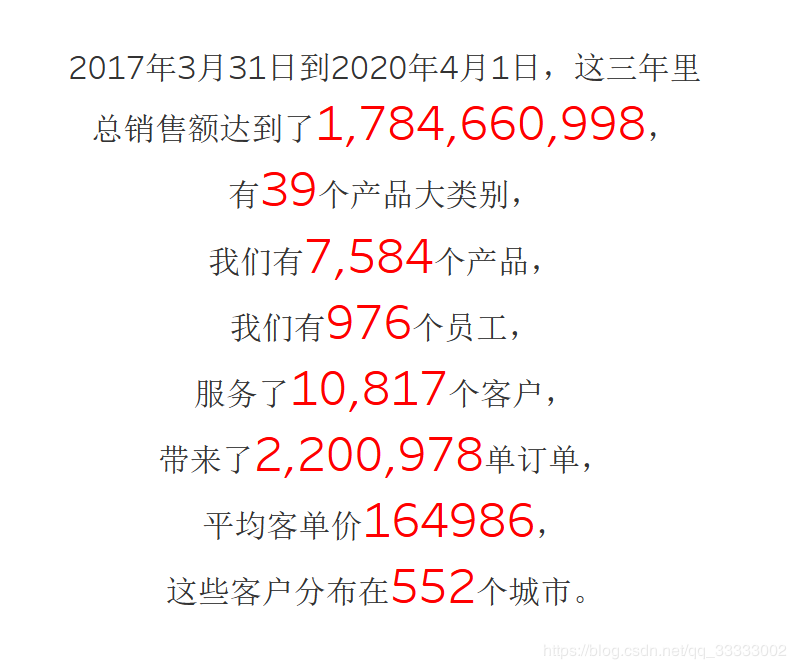### 4.2 时间角度

#### 4.2.1  年销售额情况

2017年只有前9个月的销售额，2020年只有前4个月的销售额。

2019年总销售额达到718306933，环比2018年的680191151，增长5.6%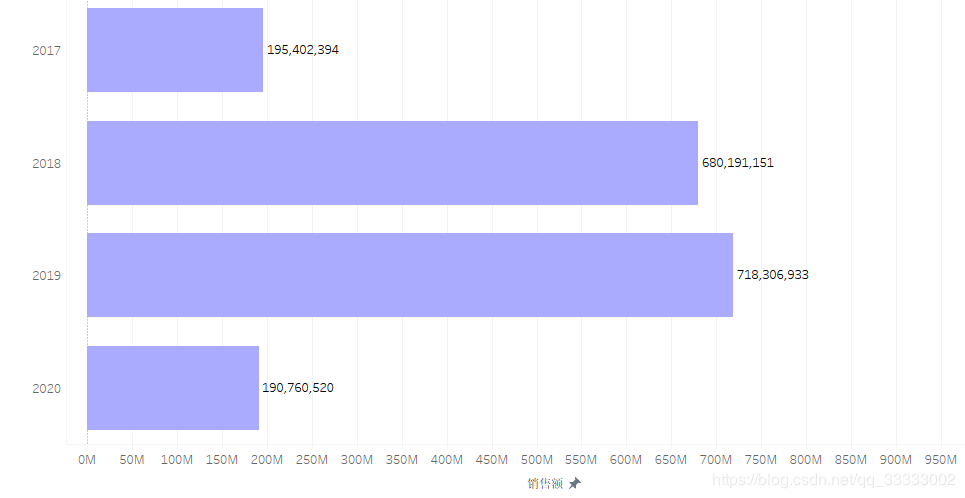#### 4.2.2  季度的销售额情况

2017第二季度开始到2018年底订单量成直线式上涨，2019年较平稳。

2017年该连锁店出于疯狂生长期，订单量、销售额均呈现直线上升趋势。

2019年第四季度订单量：208548，销售额达到206513981，订单量、销售金额均达到历史峰值。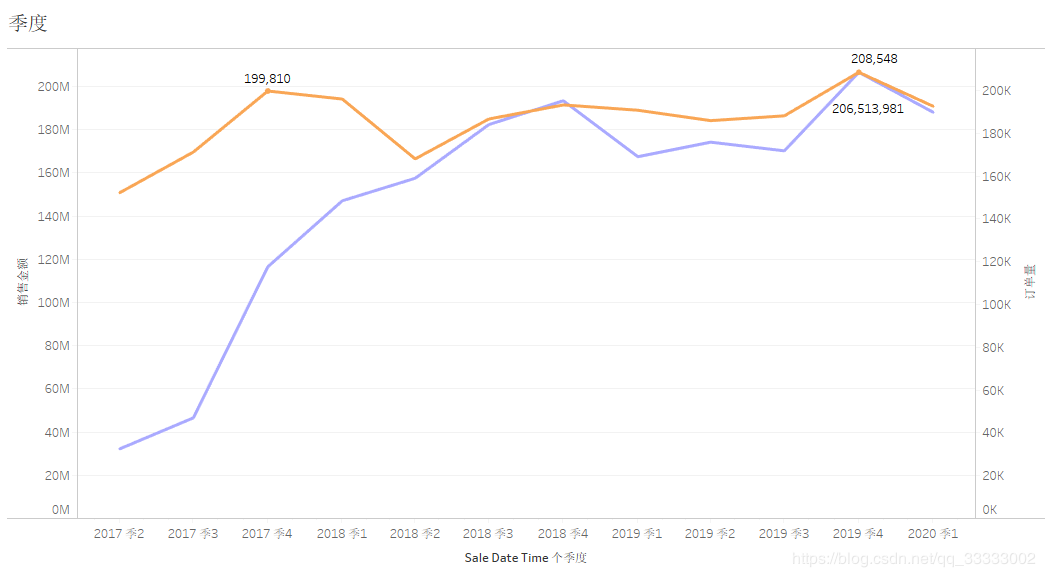#### 4.2.3 月的销售情况

2017年各月份的销售金额，呈上涨趋势，其中17年下半年上涨趋势较明显，18、19年呈现较稳定的状态；结合各月份，连锁店的数量。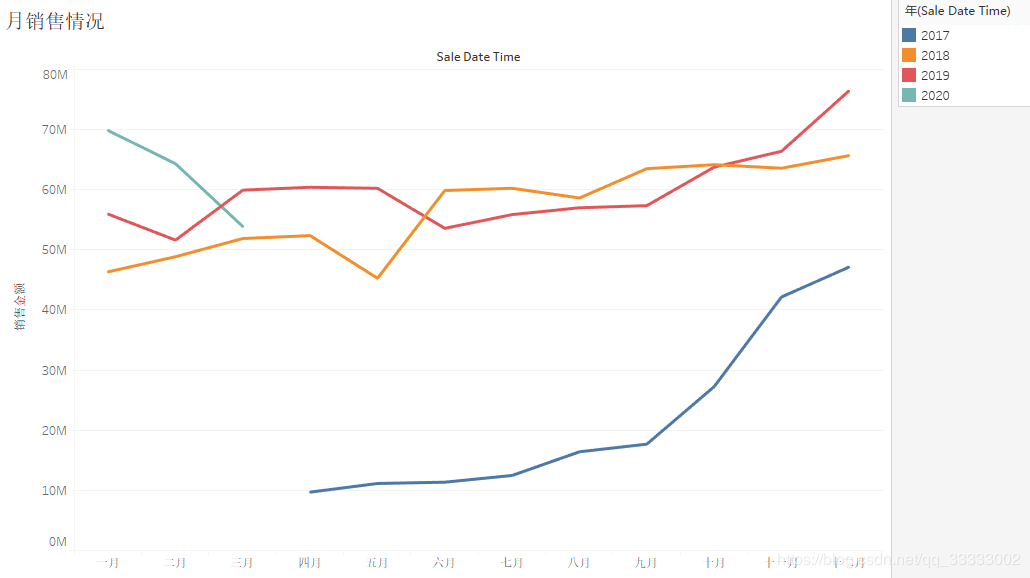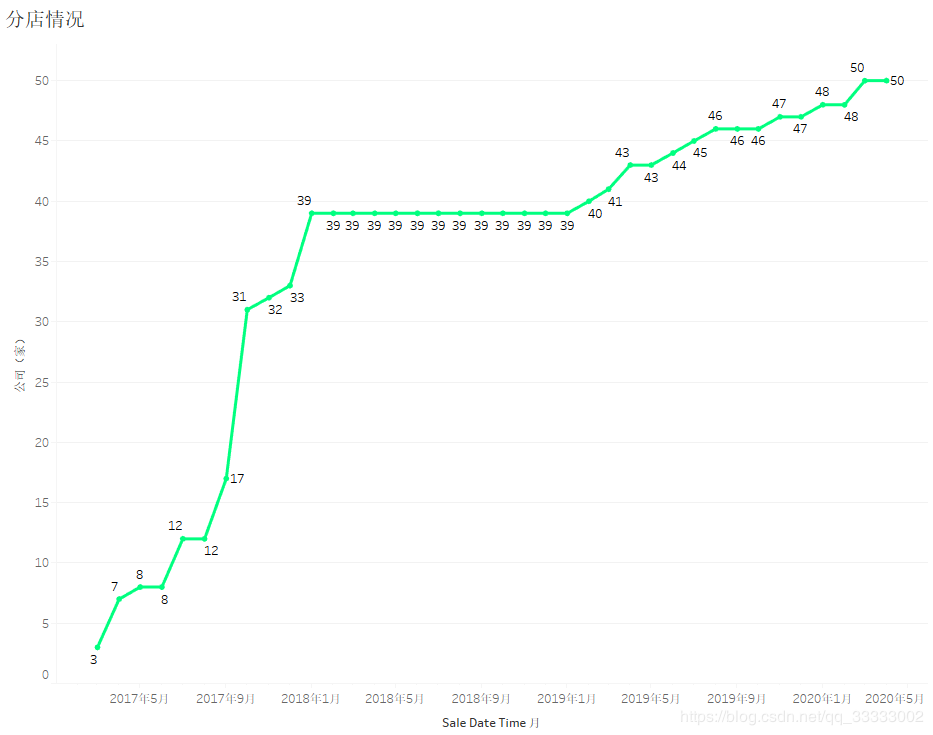#### 4.2.4 周的销售情况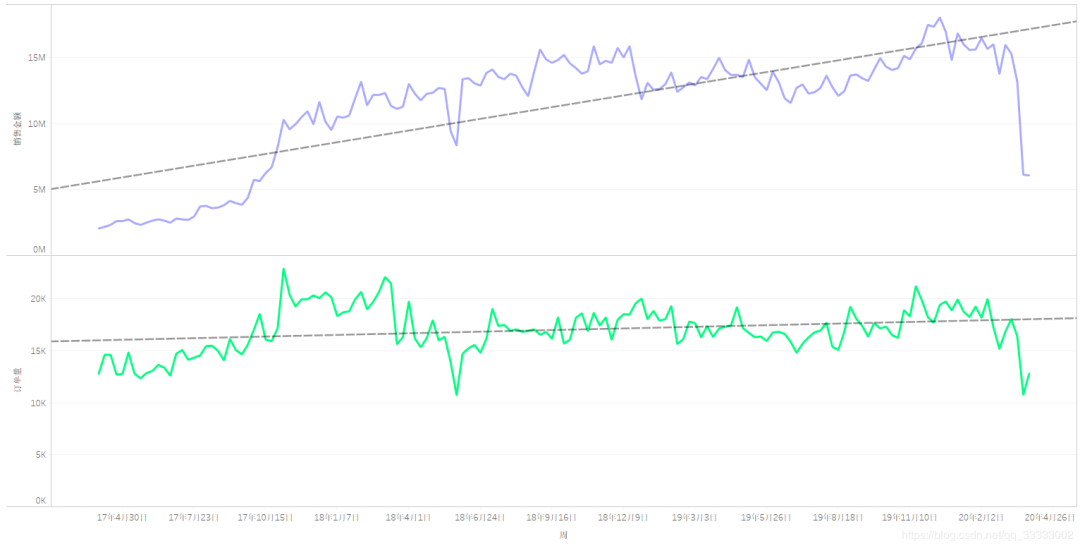#### 4.2.5 日的销售情况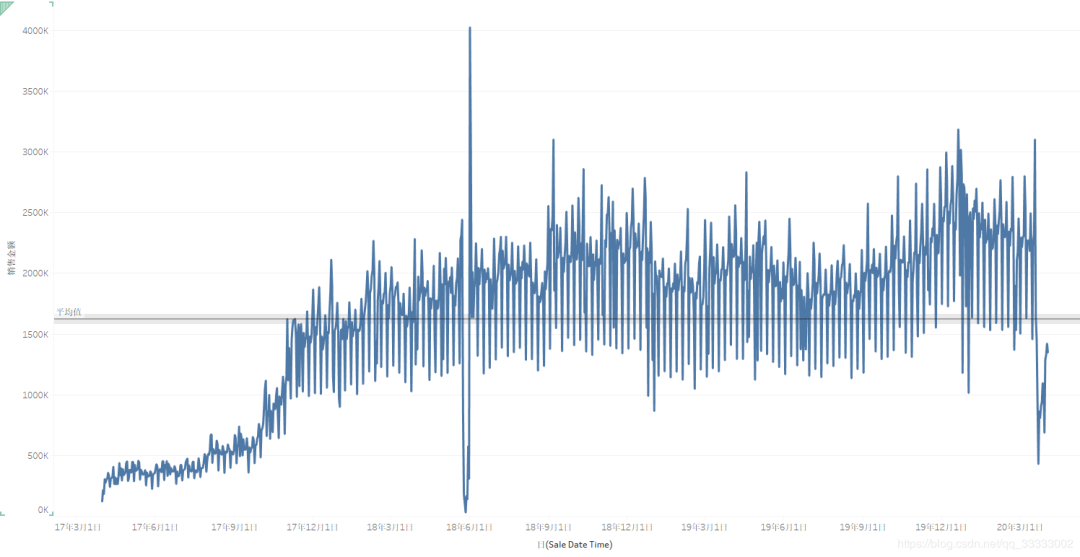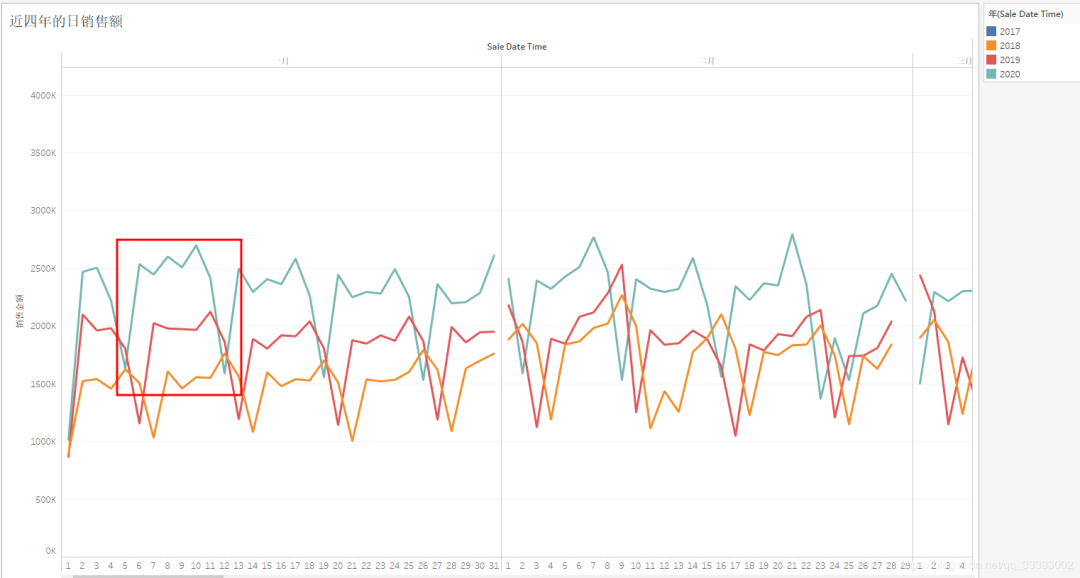#### 4.2.6 近四年的日UV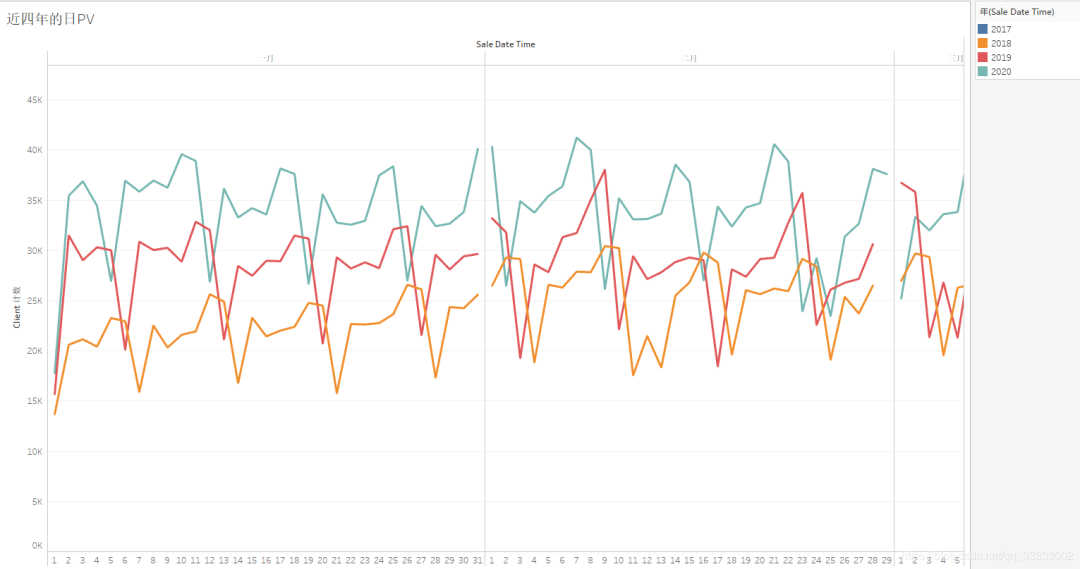#### 4.2.7 工作日的销售情况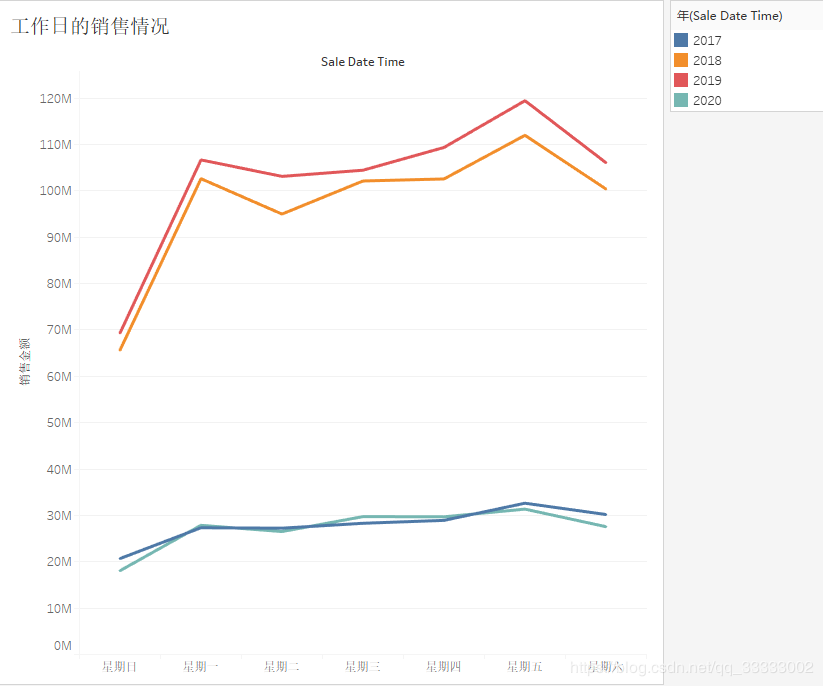#### 4.2.7 工作日的订单量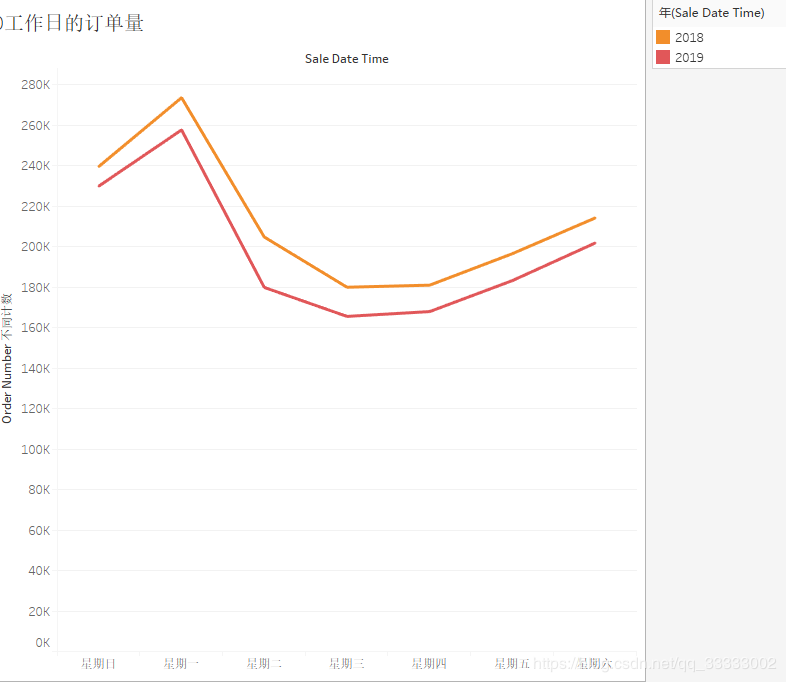#### 4.2.8 时间段的销售金额、订单量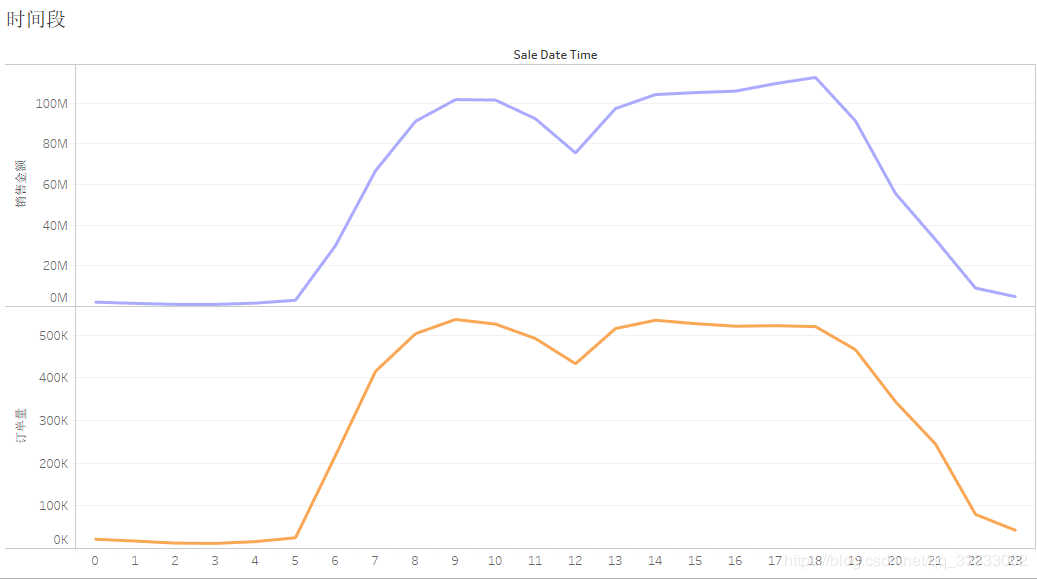#### 4.2.9 工作日的销售权重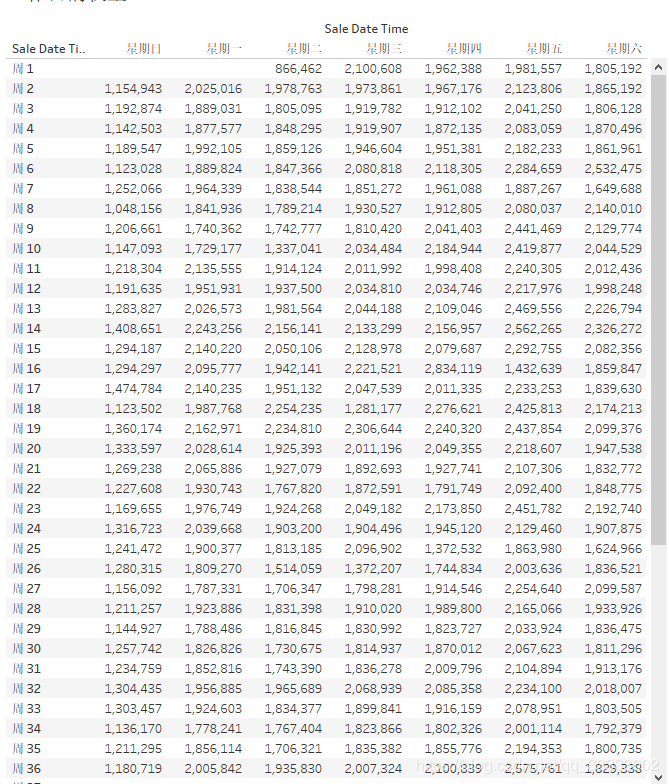1. 全年周日的平均值=全年的周日的总销售额/全年周日的天数，其他工作日类推。

2. 挑选1中计算到的最小值

3. 权重=某个工作日的平均值 / 2中选出的最小值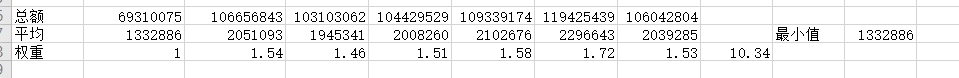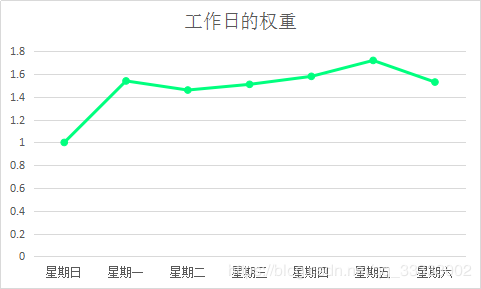### 4.2.10 销售预测按日

# 将销售时间设置成索引
data.set_index('Sale Date Time', inplace=True, drop=True)
# 将数据重新整理成以天来统计每天销售额的汇总
day_data = data.resample('d').sum()['Total']
day_data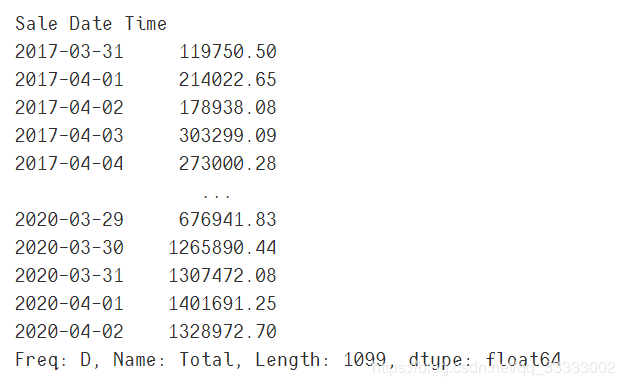# 挑选2019年1月1日到2020年2月29的数据
train_day_data = day_data[day_data.index >= '2019-01-01']
train_day_data = train_day_data[train_day_data.index <= '2020-02-29']
# 保存数据到Excel
train_day_data.to_excel('./日销售数据.xlsx')


# 读取数据
# 重新命名列
data.rename(columns={'Sale Date Time': 'date1'}, inplace=True)
data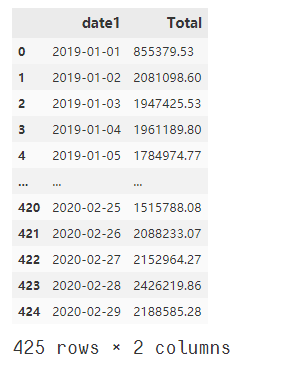# 将销售额进行缩放，预测的只是大概的值，不可能太精确，这里直接根据数据的情况，以10万作为基本的单位。
data['Total'] = round(data['Total'] / 100000, 4)



import matplotlib.pyplot as plt

plt.rcParams['font.sans-serif'] = 'SimHei'
plt.rcParams['axes.unicode_minus'] = False
%matplotlib inline

# 时序图
plt.figure(figsize=(18, 8), dpi=256)
data['Total'][:-30].plot()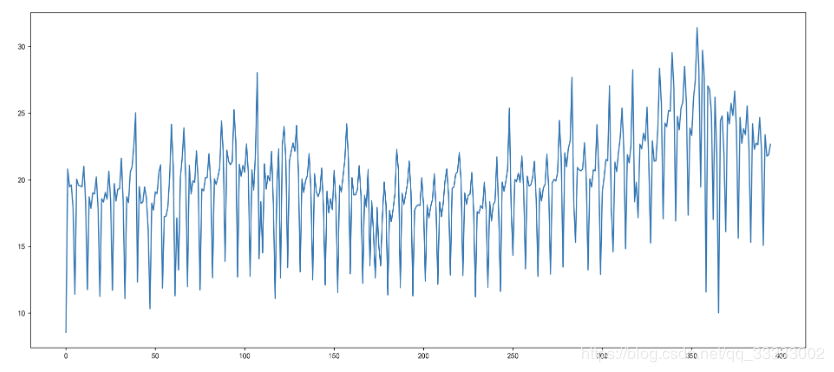# 自相关图
from statsmodels.graphics.tsaplots import plot_acf
plot_acf(data['Total'][:-30])
plt.figure(figsize=(18, 8), dpi=256)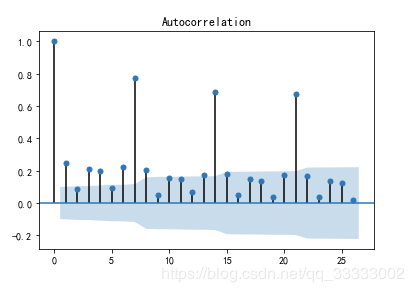# 偏自相关图
from statsmodels.graphics.tsaplots import plot_pacf
plot_pacf(data['Total'][:-30])
plt.figure(figsize=(18, 8), dpi=256)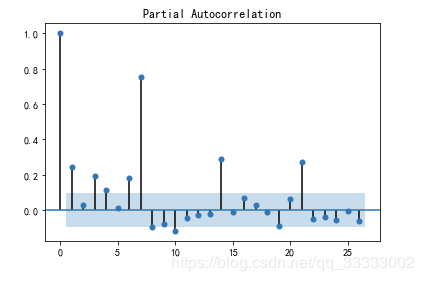# 单位跟检验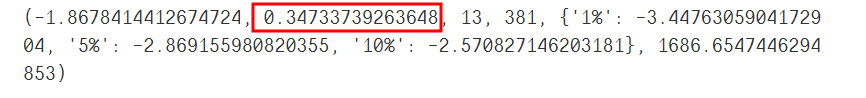# 一阶差分
D_data = data['Total'][:-30].diff().dropna()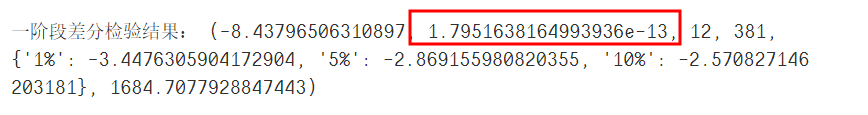from statsmodels.stats.diagnostic import acorr_ljungbox
print('白噪声检验结果：', acorr_ljungbox(D_data, lags=1))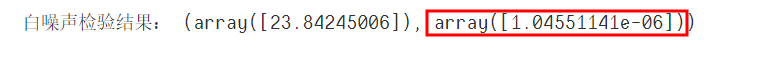from statsmodels.tsa.arima_model import ARIMA
from datetime import datetime
from itertools import product

# 设置p阶，q阶范围
# product p,q的所有组合
# 设置最好的aic为无穷大
# 对范围内的p,q阶进行模型训练，得到最优模型

ps = range(0, 5)
qs = range(0, 5)
parameters = product(ps, qs)
parameters_list = list(parameters)

best_aic = float('inf')
results = []
for param in parameters_list:
try:
model = ARIMA(data['Total'][:-30], order=(param, 1, param)).fit()
except ValueError:
print("参数错误：", param)
continue
aic = model.aic
if aic < best_aic:
best_model = model
best_aic = model.aic
best_param = param
results.append([param, model.aic])
results_table = pd.DataFrame(results)
results_table.columns = ['parameters', 'aic']
print("最优模型", best_model.summary())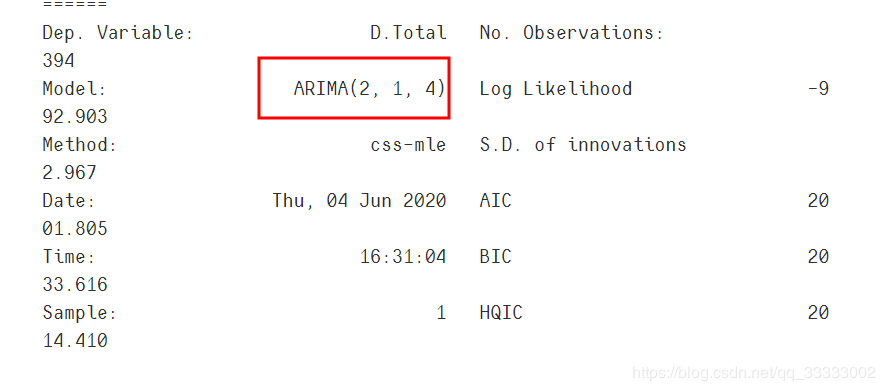best_model.forecast(30)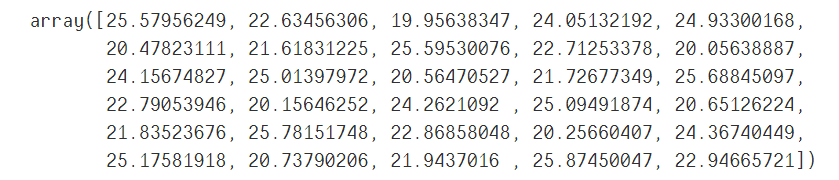from sklearn.metrics import mean_absolute_error
# pred_y 预测值
# test_y 实际值
pred_y = best_model.forecast(30)
test_y = data['Total'][-30:].values
mean_absolute_error(test_y, pred_y)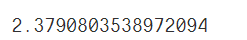plt.figure(figsize=(14, 7), dpi=256)
plt.plot(data['date1'][-30:], test_y, label='实际')
plt.plot(data['date1'][-30:], pred_y, label='预测')
plt.xticks(data['date1'][-30:], rotation=70)
plt.legend(loc=3)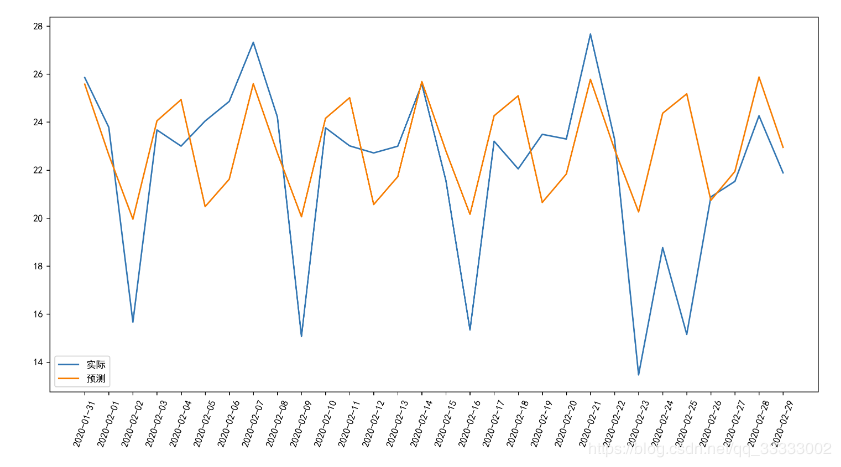### 4.3 用户角度

#### 4.3.1 用户城市分布

10.98%的用户集中在Agirrie这个城市，用户居住城市相对较分散。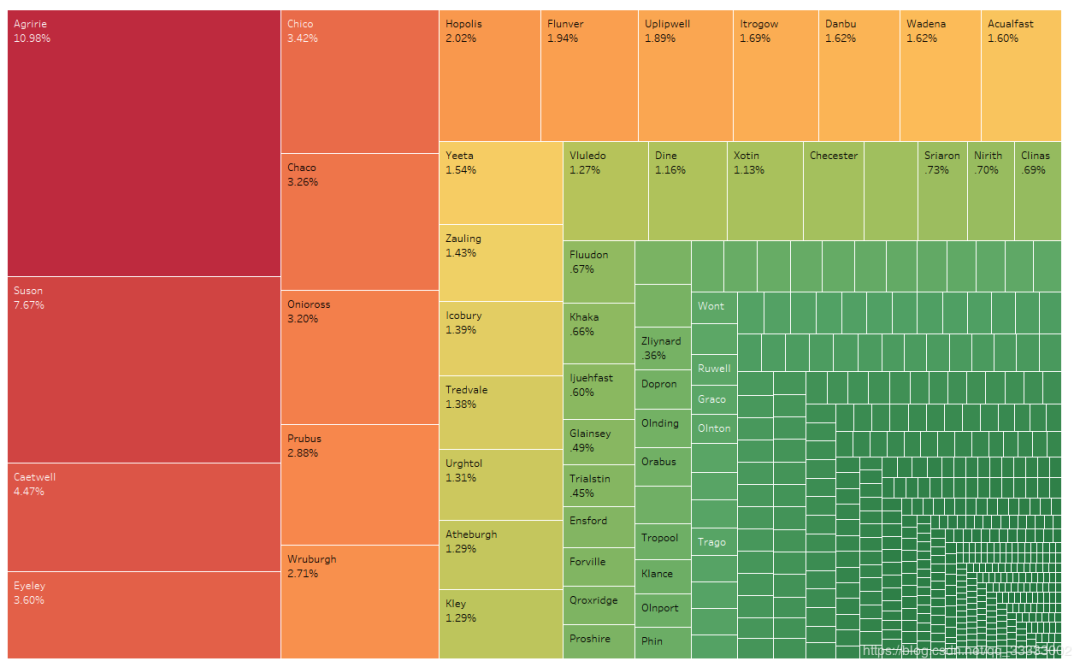#### 4.3.2 用户购买金额，购买次数前10的用户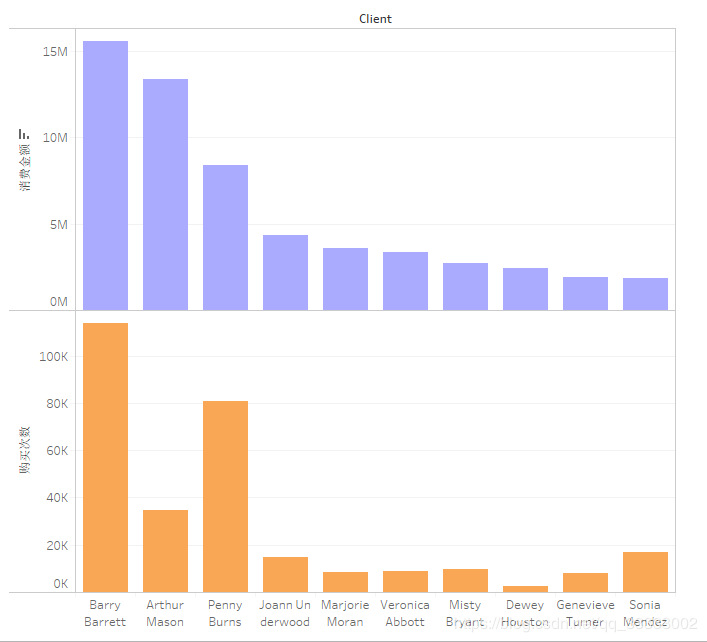#### 4.3.3 复购率

##### 总体复购率：

# 计算每个客户的购买次数，这里使用了nunique(),统计不同订单号的个数
client_data = data.groupby('Client').nunique()['Order Number']
# 重命名列
client_data = client_data.reset_index().rename(columns={'Order Number':
'user_num'})
client_data.sort_values('user_num', ascending=False)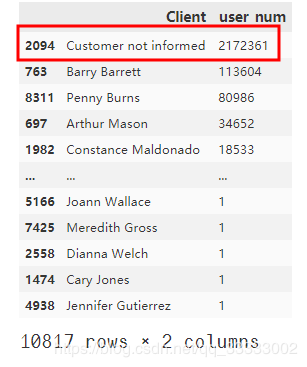# 总复购率
print('总复购率:',
round(
(len(client_data[client_data['user_num'] > 1])-1) /
(len(client_data) - 1), 4) * 100, "%")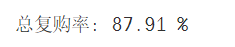##### 一个月内复购率

# 这里的销售时间是datetime格式，增加个辅助列，转换成2017-01这样的年月显示
def parse_year_month(x):
if x.month >= 10:
return str(x.year) + "-" + str(x.month)
else:
return str(x.year) + "-0" + str(x.month)
data['year_month'] = data['Sale Date Time'].apply(parse_year_month)



y_m_data = data.groupby(['year_month',
'Client']).nunique()['Order Number'].reset_index()
y_m_data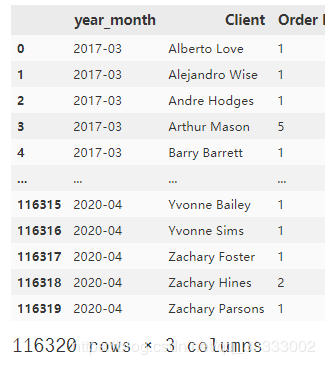# 保存临时数据，用于构建每月的复购率的DataFrame
month_list = []
rate_list = []
# 循环计算每个月的复购率，这里直接遍历每个月
for every_m in y_m_data['year_month'].unique():
#     获取每个月用户的购买次数的数据
temp = y_m_data[y_m_data['year_month'] == every_m]
#     print(every_m, "复购率：",
#           round((len(temp[temp['Order Number'] > 1])-1) / (len(temp) -1),4))
month_list.append(every_m)
#     选出购买次数>1的数据，获取数据的长度（用户数）- 1 再除以
# 当月的总用户数 -1
rate_list.append(round((len(temp[temp['Order Number'] > 1])-1) / (len(temp) -1), 4))
#将数据转换成DataFrame
t_1 = {'month': month_list, 'rate': rate_list}
rate_data = pd.DataFrame(t_1)
rate_data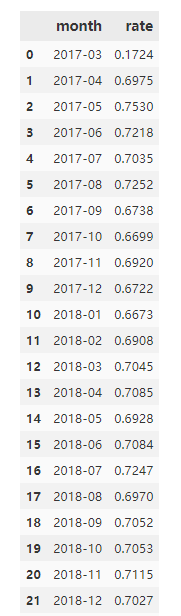rate_data.to_excel('./rate_data.xlsx', index=False)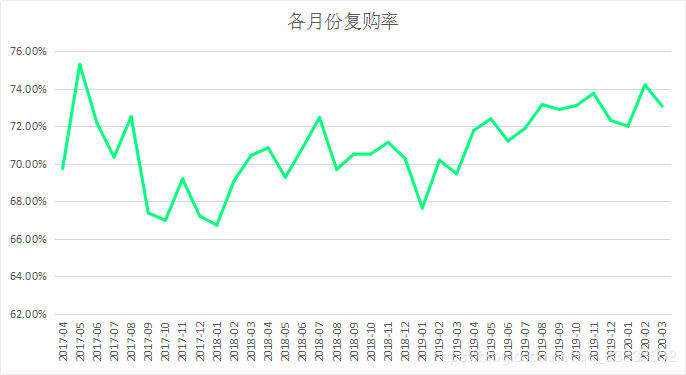#### 4.3.4 购买次数的情况

data_201804 = y_m_data[y_m_data['year_month'] == '2018-04']
data_201804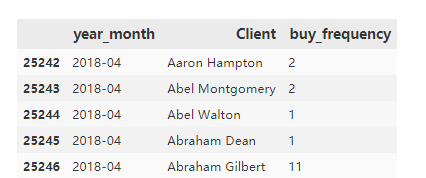# 设置数据的区间
bins = [0, 1, 2, 5, 10, 50, 100, 100000]
per_frequency.value_counts()
per_frequency.value_counts().plot(kind='bar')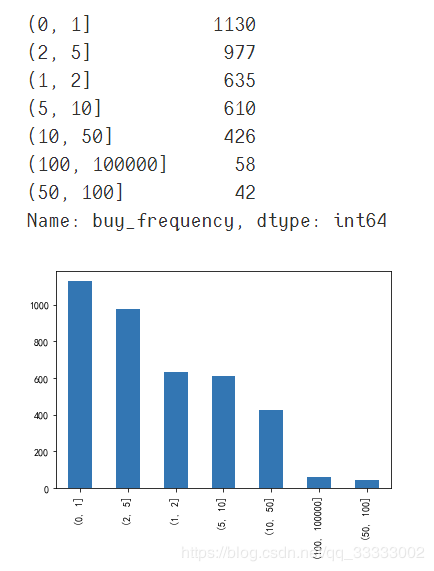#### 4.3.5 RFM模型分析用户的价值

# 按月份提取每个月用户的R、F、M值
RFM_data_all = data.groupby(['year_month',
'Client']).agg({'Order Number': 'nunique',
'Sale Date Time': 'max',
'Total': 'sum'})
RFM_data_all.reset_index(inplace=True)
# 保存一份数据，下次直接读取该数据集就可以，省时间
RFM_data_all.to_excel('RFM_data_all.xlsx', index=False)

# 提取2018年4月份的数据
RFM_data_201804  = RFM_data_all[RFM_data_all['year_month'] == '2018-04']
RFM_data_201804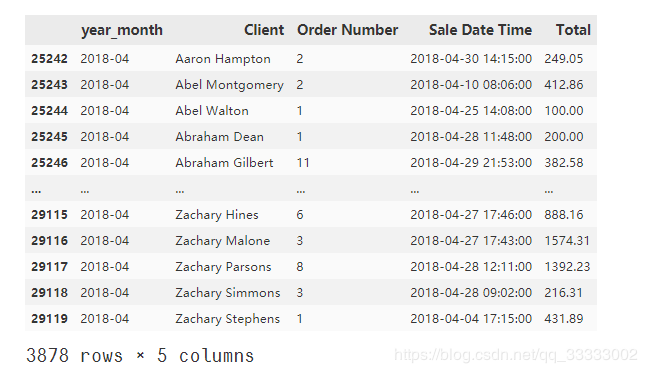# 参考时间，这里随便设置里2018-05-01 23:59:59，不让R值为0，这里的R以天作为单位
import datetime
reference_time = datetime.datetime.strptime('2018-05-01 23:59:59',
"%Y-%m-%d %H:%M:%S")
# 构建R指标
RFM_data_201804['R'] = RFM_data_201804['Sale Date Time'].apply(lambda x: (
reference_time - x).days)
# 重新命名列
RFM_data_201804.rename(columns={'Order Number': 'F', 'Total': 'M'}, inplace=True)
# 排序查看异常值
RFM_data_201804.sort_values('M', ascending=False)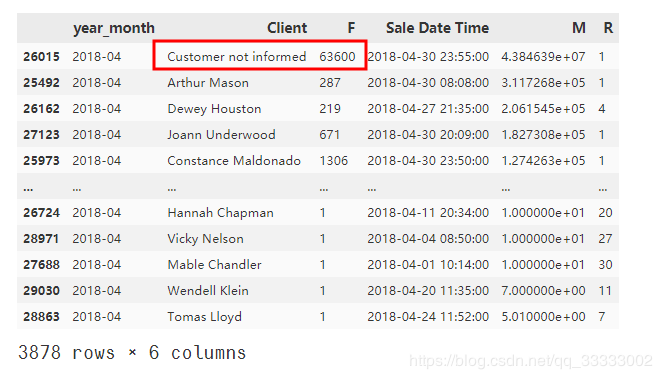RFM_data_201804.drop(index=26015, inplace=True)



RFM_data = RFM_data_201804[['R', 'F', 'M']]



from sklearn.preprocessing import StandardScaler
from sklearn.cluster import KMeans
#数据规范化
ss  = StandardScaler()
train = ss.fit_transform(RFM_data)
# 模型进行训练，这里直接聚类成5类。
kmeans_model = KMeans(n_clusters=5)
kmeans_model.fit(train)
#查看聚类中心
test = pd.DataFrame(kmeans_model.cluster_centers_, columns=['R', 'F', 'M'])
test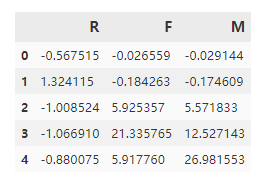RFM_data['sk5_label'] = kmeans_model.labels_



RFM_data[RFM_data['sk5_label'] == 3]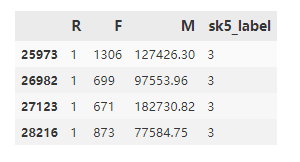RFM_data[RFM_data['sk5_label'] == 4]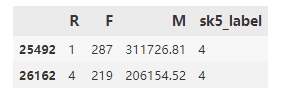Tableau实现的客户分群：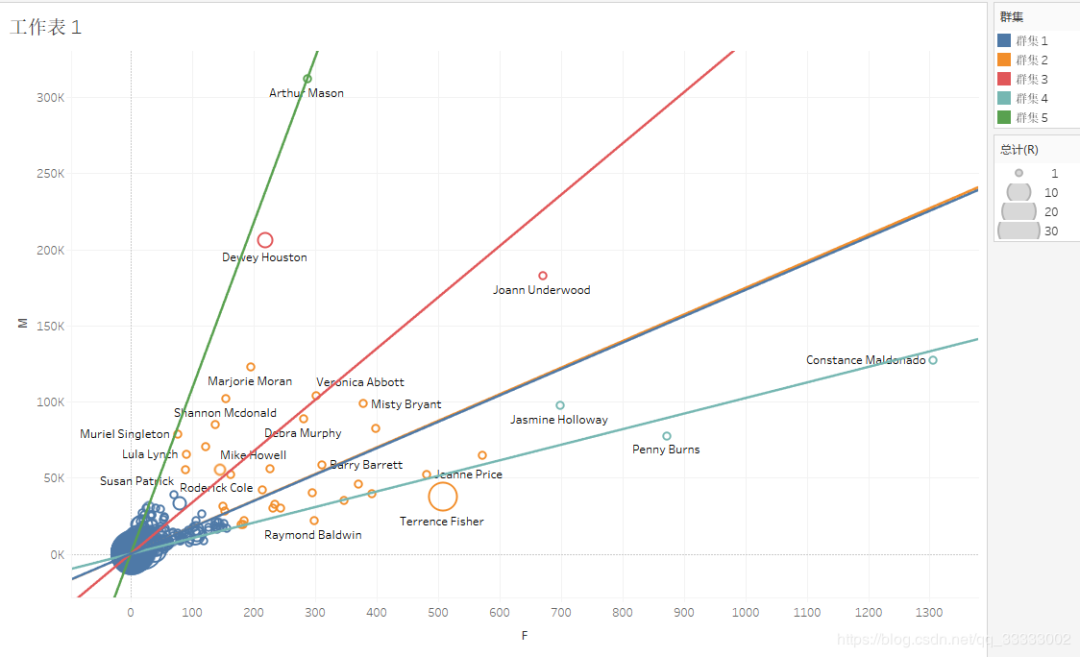#### 4.3.6 用户月留存率

# 提取每个月的用户（去重）
every_month_user = data.groupby(['year_month',
'Client']).nunique()['Order Number'].reset_index()
every_month_user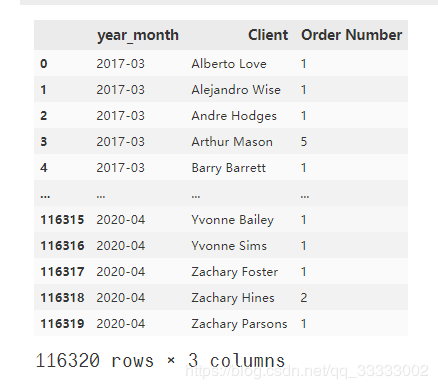# 获取每个月份的列表，循环遍历计算上个月与当前月的留存率
year_month = every_month_user['year_month'].unique()
# 保存月留存率的列表
list_month_rate = []
for i, month in enumerate(year_month):
#     计算上个月与当前月的留存率
if i>= 1:
#         获取当前月的用户（上面groupby已去重）
this_month_client = every_month_user[every_month_user['year_month'] == month]['Client']
#        获取上个月的用户（上面groupby已去重）
previous_month_client = every_month_user[every_month_user['year_month'] == year_month[i-1]]['Client']
#         计算留存率，这里用的是上个月与当前月用户的交集个数/上个月的用户数（去重）
rate = round(len (set(this_month_client) & set(previous_month_client))
/ len(previous_month_client),2)
#         用列表保存数据，并构建DataFrame用户绘图
b = [month, rate]
list_month_rate.append(b)
# 构建DataFrame
rate_data = pd.DataFrame(list_month_rate, columns=['year_month', 'rate'])
rate_data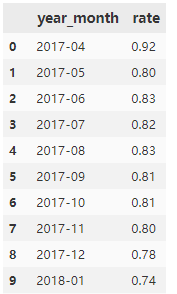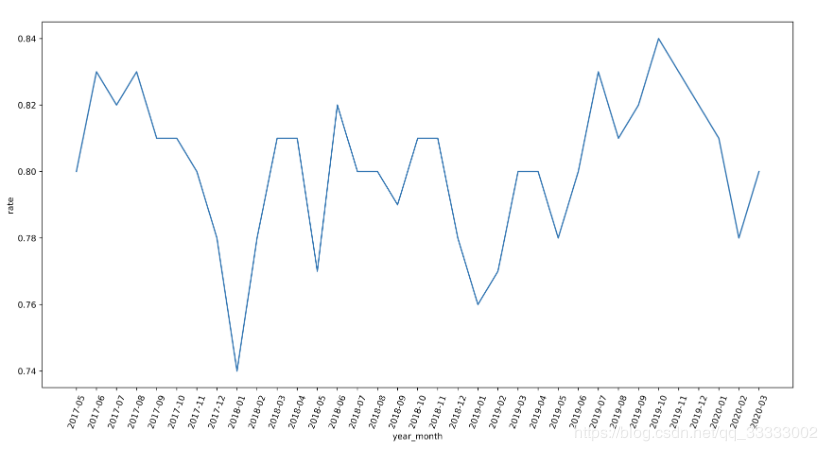### 4.4 产品角度

#### 4.4.1 销售额，订单量前10的销售产品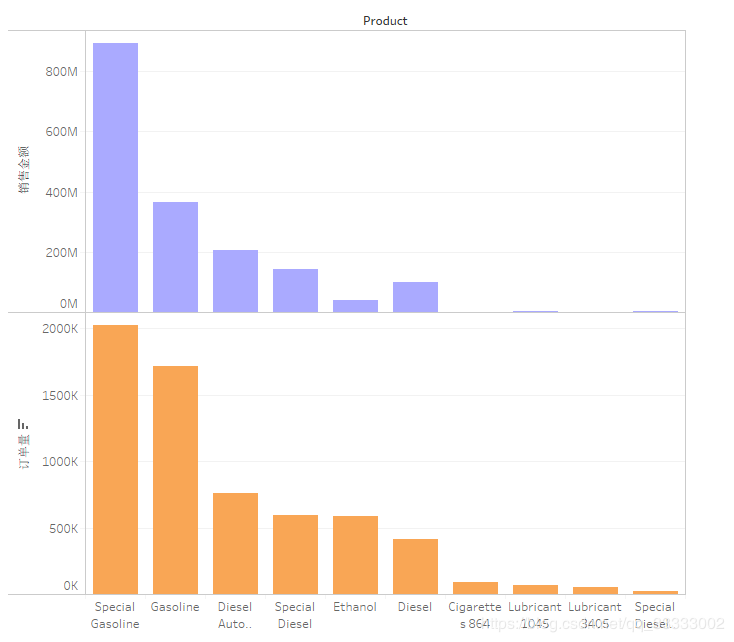#### 4.4.2 产品分类（聚类分析）

month_product_data = data.groupby(['year_month',
'Product']).agg({
'Product Cost': 'mean',
'Order Number': 'nunique',
'Total': 'sum'
}).reset_index()

# 重命名
month_product_data.rename(columns={'Product Cost': 'C', 'Order Number': 'F',
'Total': 'M'}, inplace=True)
# 导出数据到Excel,结合Tableau一起分析下。
month_product_data.to_excel('./month_product_data.xlsx', index=False)
# 选择2018年4月的数据
# 这里只取一个月的产品进行聚类
month_product_201804 = month_product_data[month_product_data['year_month'] == '2018-04']
month_product_201804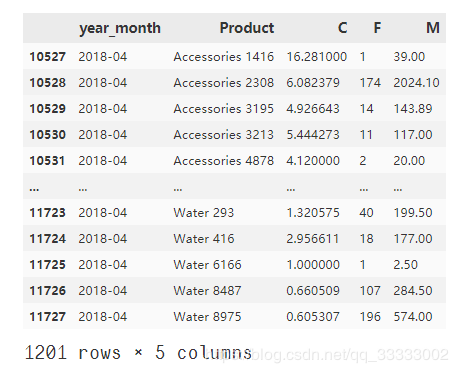# 导入包
from sklearn.preprocessing import StandardScaler
from sklearn.cluster import DBSCAN
ss = StandardScaler()
# 获取需要的数据
X = month_product_201804[['C', 'F', 'M']]
# 数据规范化
train_X = ss.fit_transform(X)
# 设置聚类数4个
dbscan_model = DBSCAN(min_samples=4)
# 模型训练
dbscan_model.fit(train_X)
# 将聚类的结果合并到原数据集上。
month_product_201804['labels'] = dbscan_model.labels_
# 查看聚类的分布情况
month_product_201804['labels'].value_counts()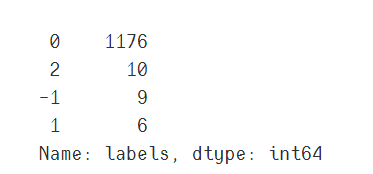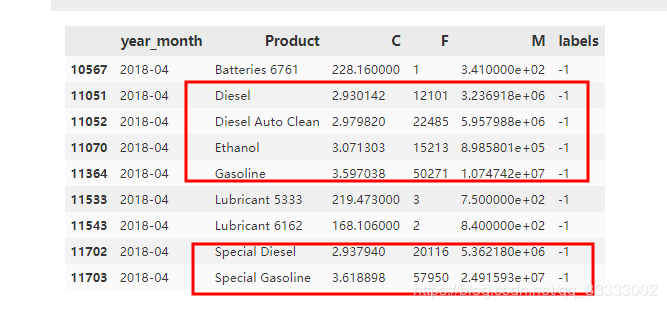A类（上图和下图截红框）：成本低，订单量多，购买金额多的，这类属于重点开发的产品。

B类：成本高，订单量少，购买金额少的，这类属于低价值的产品，应该砍掉。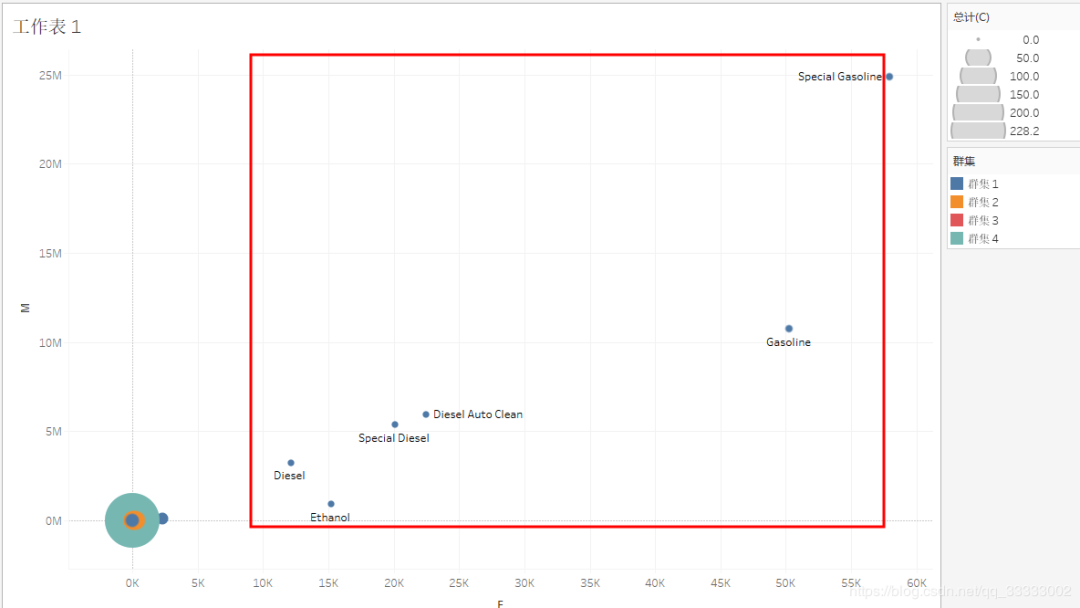Tableau排除上图截红框的一个教特殊的产品再进行产品的聚类。

##### 针对群集4：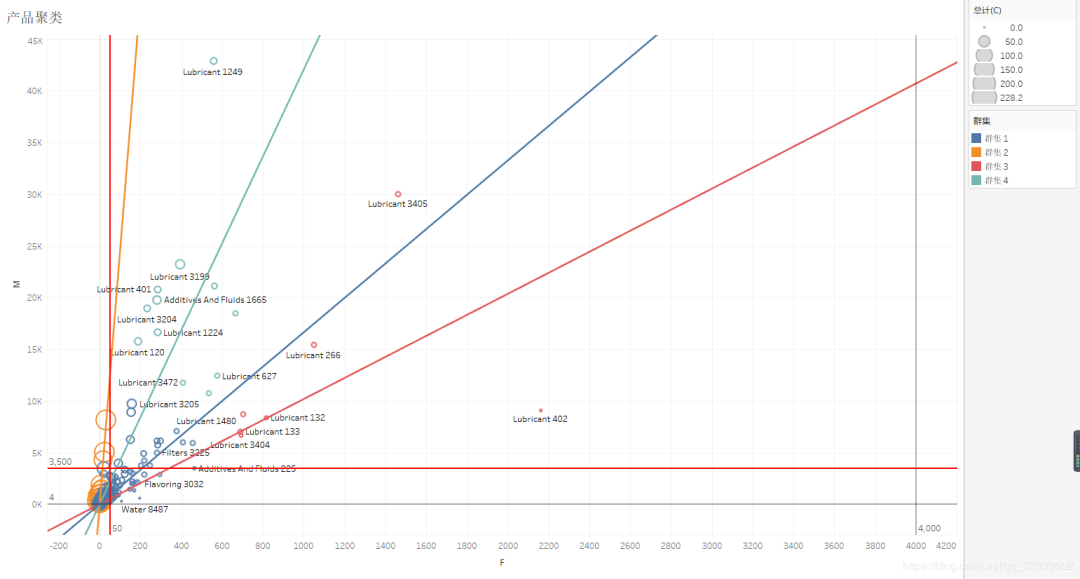#### 4.4.3 产品销售额情况及总体的占比（帕累托最优）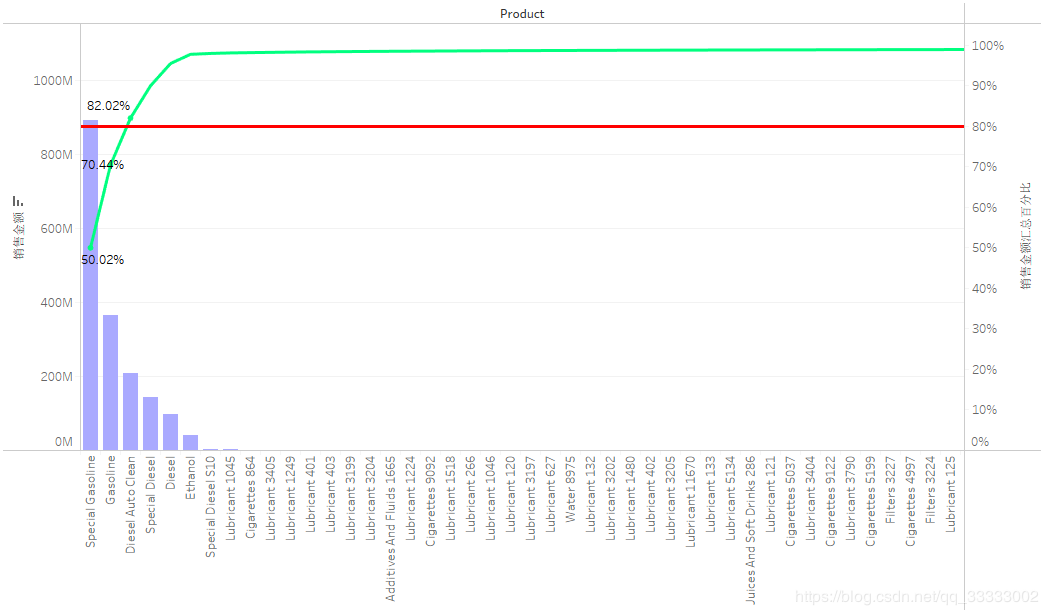#### 4.4.4 产品的成本分布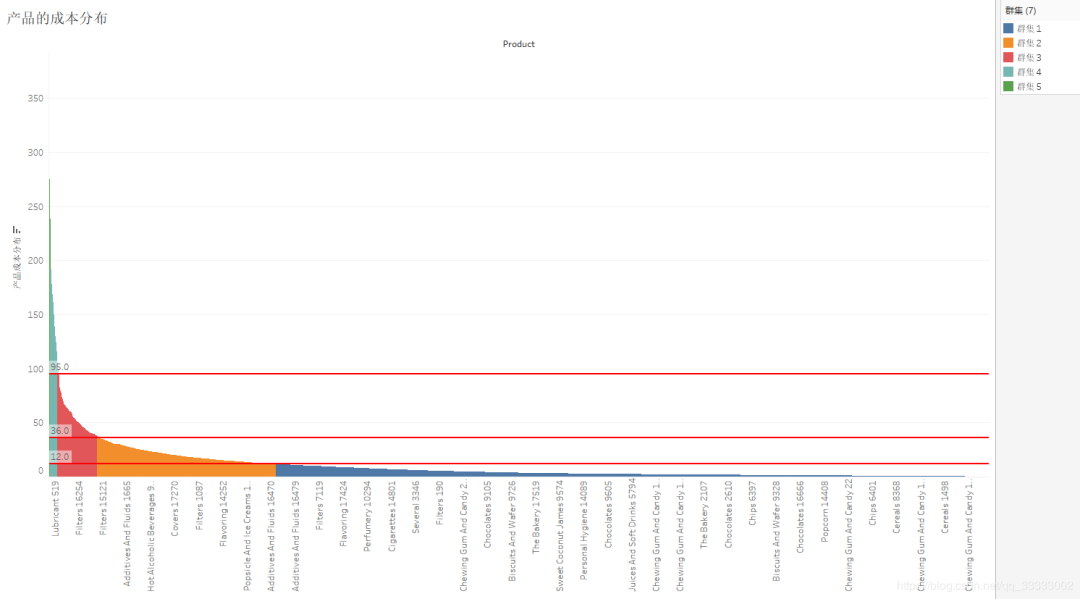#### 4.4.5 跟目录下的各个产品数

Filters产品类别下的产品数最多，达到933。其次是839Chewing Gum And Candy。最少的产品数的是类别Extinguisher，只有6个。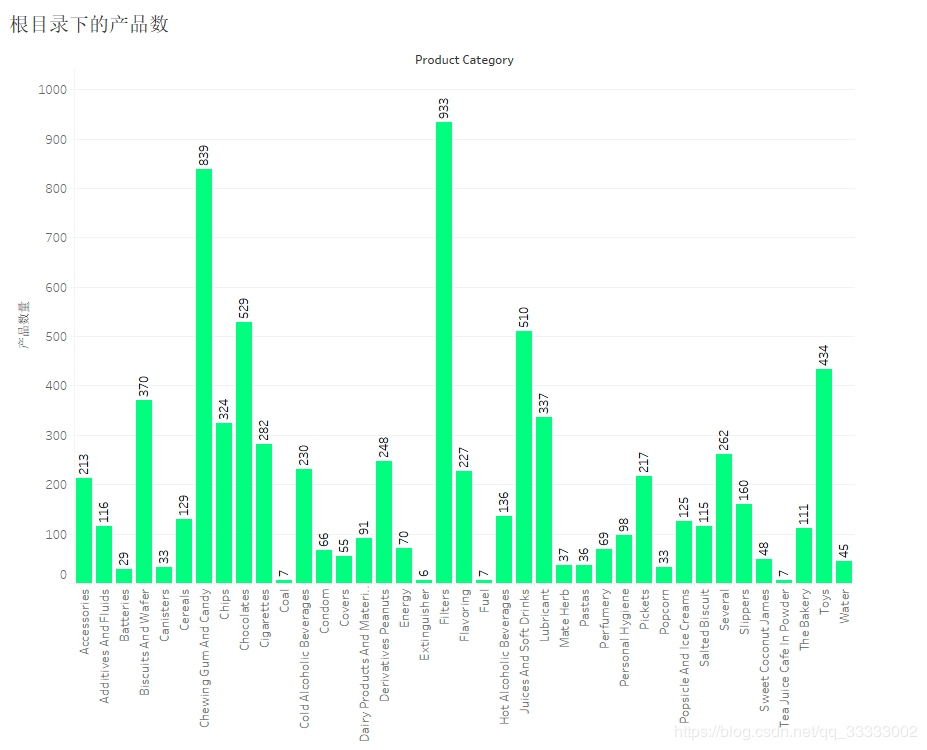## 支付方式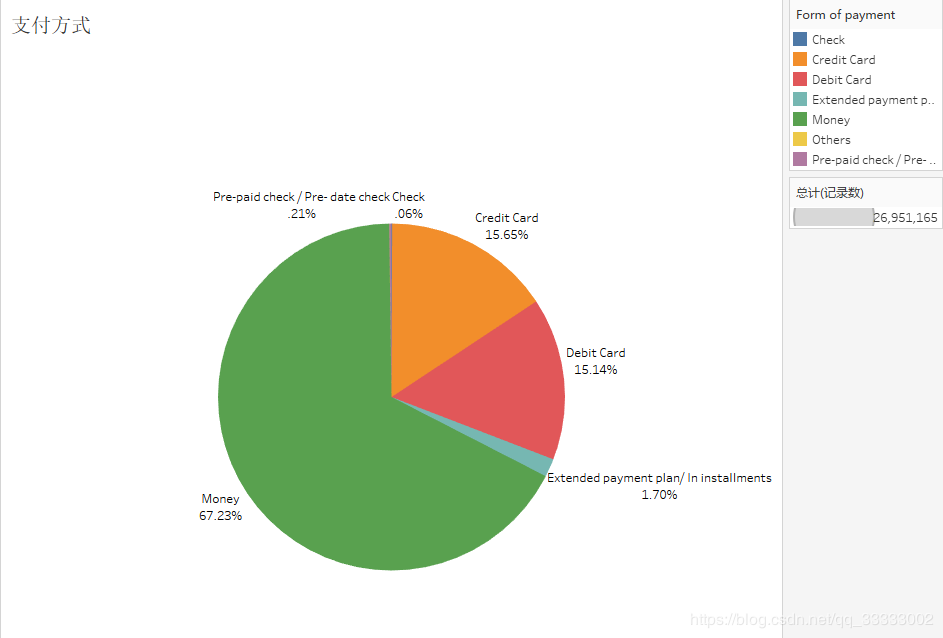## 总结

##### 6.9 近七成的用户选择现金支付。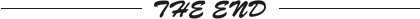更多精彩推荐
☞去世这天是她的生日，全球首位女性图灵奖得主 Frances Allen 的传奇人生
☞华为云 GaussDB 数据库，会是新的国产之光吗？
☞小米十年，雷军的一往无前
☞用Bi-GRU语义解析，实现中文人物关系分析
☞CPU：别再拿我当搬砖工！
☞DeFi升空助推器：收益耕作者「Yield Farming」


05-16649212-14
08-036700
09-071527